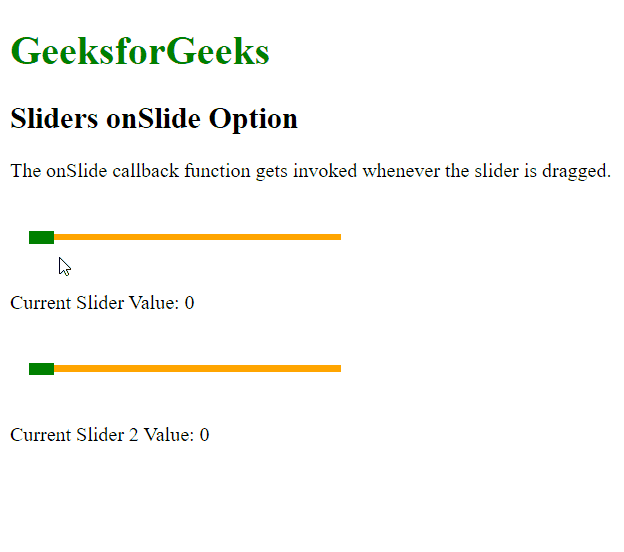Related Articles
script.aculo.us Sliders onSlide Option
• Last Updated : 30 Dec, 2020

The script.aculo.us library is a cross-browser library that aims at improving the user interface of a website. The Slider controls are thin tracks that allow the user to input values. It is done by defining a range of values that can be selected by the user by dragging the handle to the appropriate value.

The onSlide option is used to specify a callback function that would be invoked whenever the slider is moved by dragging. The current value of the slider would be passed as a parameter to the function.

Syntax:

`{ onChange: function }`

Parameters: This option has a single value as mentioned above and described below:

• function: This is a callback function that would be invoked whenever the slider is being moved by dragging.

The below example illustrates the use of this option.

Example:

## HTML

 ```<``html``>``<``head``>``  ````  ``<``script` `type``=``"text/javascript"``          ``src``=``"prototype.js"``>``  ````  ``<``script` `type``=``"text/javascript"` `          ``src``=``"scriptaculous.js?load = slider"``>``  ```` ` `  ````  ``<``style` `type``=``"text/css"``>``    ``.track {``      ``width: 250px;``      ``background-color: orange;``      ``height: 5px;``      ``position: relative;``    ``}`` ` `    ``.track .handle {``      ``width: 20px;``      ``height: 10px;``      ``background-color: green;``      ``cursor: move;``      ``position: absolute;``      ``top: -2px;``    ``}`` ` `    ``.pad {``      ``padding: 25px 15px;``    ``}``  ``````<``body``>``   ` `<``p``>``  ``<``h1` `style``=``"color: green;"``>``    ``GeeksforGeeks``  ````  ``<``h2``>Sliders onSlide Option``   ` `<``p``>``    ``The onSlide callback function ``    ``gets invoked whenever the slider``    ``is dragged.``  ```` ` `  ``<``div` `class``=``"pad"``>``    ``<``div` `id``=``"track-hor"` `class``=``"track"``>``      ``<``div` `id``=``"handle-hor"` `class``=``"handle"``>``      ````    ````  ```` ` `   ` `<``p``>Current Slider Value: ``    ``<``span` `id``=``"out"``>0``  ```` ` ` ` `  ``<``div` `class``=``"pad"``>``    ``<``div` `id``=``"track-hor2"` `class``=``"track"``>``      ``<``div` `id``=``"handle-hor2"` `class``=``"handle"``>``      ````    ````  ```` ` `   ` `<``p``>Current Slider 2 Value: ``    ``<``span` `id``=``"out2"``>0``  ```` ` ` ` `  ``<``script` `type``=``"text/javascript"``>`` ` `    ``// Initialize the slider``    ``let slider = new Control.Slider('handle-hor',``      ``'track-hor', {``       ` `      ``// Define the range``      ``range: \$R(1, 100),`` ` `      ``// Define the callback function to be``      ``// invoked when the slider is being``      ``// dragged``      ``onSlide: function (currValue) {``        ``document.querySelector("#out")``                  ``.textContent = currValue;``      ``}``    ``});`` ` `    ``// Initialize the slider``    ``let slider2 = new Control.Slider('handle-hor2',``      ``'track-hor2', {``       ` `      ``// Define the range``      ``range: \$R(1, 100),``      ``values: [1, 5, 10, 50, 80, 100],`` ` `      ``// Define the callback function to be``      ``// invoked when the slider is being``      ``// dragged``      ``onSlide: function (currValue) {``        ``document.querySelector("#out2")``                  ``.textContent = currValue;``      ``}``    ``});``  ```````

Output:Attention reader! Don’t stop learning now. Get hold of all the important DSA concepts with the DSA Self Paced Course at a student-friendly price and become industry ready.

My Personal Notes arrow_drop_up